# HC Verma Solutions Class 11 Chapter 5 Newton’s Law of Motion

HC Verma Solutions class 11 chapter 5 – Newton’s Law of Motion is one of the key chapters to study in class 11 physics. Learning the different topics covered in this chapter will be incredibly helpful in not only scoring the highest amount of marks in the exam but it will also be useful in preparing for JEE Advanced exams. If a student is appearing for competitive exams, he/she should be prepared for any kind of questions that may be asked during the examination. By referring to HC Verma Vol 1 solutions for chapter 5 – Newton’s Law Of Motion students will basically learn about the different laws of motion as defined by the popular physicists Sir Isaac Newton. In this chapter, students will also be learning about interesting topics such as mathematical formulas involving laws of motion, free body diagram, force extended and force retracted, uniform motion, tension, etc. The solutions provided here will serve as a great reference tool for students who are using the HC Verma book to study for their exams.

1. First Law of Motion
2. Second Law of Motion
3. Working with Newton’s First and Second Law
4. Newton’s Third Law of Motion
5. Pseudo Forces
6. The Horse and the Cart
7. Inertia

## Class 11 Important Questions In Chapter 5

1. You are sitting in a car which is driven by your dad. The brakes are suddenly applied and as a result, you are pushed forward. Can you explain who pushed you forward or what made you go in that direction?
2. Skydivers generally jump from an aeroplane and free fall towards the earth’s surface for some time with some acceleration. However, when the parachute opens the speed decreases. How does the action of the parachute check the acceleration? Explain.
3.  What can you tell about an elevator when the tension in the cable supporting is equal to its weight;
(a) moving down with uniform speed
(b) moving down with increasing speed
(c) moving up with a uniform speed
(d) moving up with an increasing speed
4. A car moving along a horizontal road experiences a certain acceleration due to a force exerted by
(a) the road (b) the engine (c) the road (d) the driver.
5. What conclusion can you draw when a person says that he/she has measured the acceleration of an object to be non-zero when there was no force acting on the object.

## HC Verma Solutions Vol 1 Newton’s Law Of Motion- Chapter 5

Question 1: A block of mass 2 kg placed on a long frictionless horizontal table is pulled horizontally by a constant force F. It is found to move 10 m in the first two seconds. Find the magnitude of F.

Solution:

Here, Time = t = 2s , distance = d = 10 m, mass = m = 2 kg and initial velocity = u = 0 m/s

We know,

s = ut + 1/2 at2 [equation of motion]

on substituting the values, we have

10 = 1/2 x a x 4 = 2a

=> a = 5 m/s2

Now, the force = F = ma = 25 = 10N

Question 2: A car moving at 40 km/h is to be stopped by applying brakes in the next 4.0 m. If the car weighs 2000 kg, what average force must be applied on it?

Solution:

Here, initial velocity = u = 40 km/h or 11.12 m/s

mass of the car = 2000 kg

Distance = s = 4m

We know, 2as = v2 – u2

[equation of motion ]

8a = 0 – 11.122 = -123.65

=> a = 15.45 m/s2

Now, the force = F = ma = 2000 x 15.45 = 3.1 x 104 N

Question 3: In a TV picture tube electrons are ejected from the cathode with negligible speed and reach a velocity of 5 × 106 m/s in travelling one centimeter. Assuming straight line motion, find the constant force exerted on the electron. The mass of the electron is 9.1 × 10-31 kg.

Solution:

From given information’s:

initial velocity = u = 0 m/s

Final velocity = v = 5 × 106 m/s

mass of the electron= 9.1 × 10-31 kg

Distance = s = 1 cm

We know, 2as = v2 – u2

[equation of motion]

2a x 0.01 = (5 × 106) 2 – 0 = 25 x 1012

=> a = 12.5 x 1014 m/s2

Now, the force = F = ma = 9.1 × 10-31 x 12.5 x 1014 = 1.1 x 10-15N

Question 4: A block of mass 0.2 kg is suspended from the ceiling by a light string. A second block of mass 0.3 kg is suspended from the first block through another string. Find the tensions in the two strings. Take g = 10 m/s2.

Solution: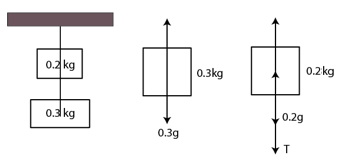The first string is being stretched due to the weight of both the blocks.

The tension in the first string = 1st block weight + 2nd block weight

= 0.2 x 10 + 0.3 x 10 = 5N

Second string is being stretched due to the weight of the second block

Tension in the first string = weight of block 2 = 0.3 x 10 = 3N

Therefore, tension in the two strings are 5 N and 3N respectively.

Question 5: Two blocks of equal mass m are tied to each other through a light string. One of the blocks is pulled along the line joining them with a constant force F. Find the tension in the string joining the blocks.

Solution: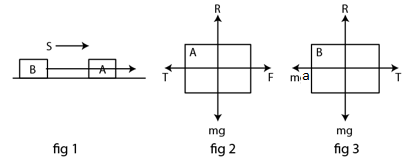We know, equation of motion can be written as:

T + ma – F = 0 …(1) or

T = ma …(2)

From (1) F = T + ma

or F = T + T = 2T [Using (2)]

=> T = F/2

Question 6: A particle of mass 50 g moves on a straight line. The variation of speed with time is shown in figure (5-E1). Find the force acting on the particle at t =2, 4 and 6 seconds.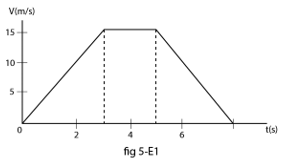Solution:

Mass = 50 g = 5×10-2 kg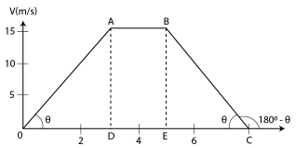Slope for region at t = 2s:

Slope of OA = tan θ = BE/EC = 15/3 =5 m/s2

Force along the motion, F = ma = 5 x 10-2 x 5 = 0.25N

Slope for region at t = 4s :

Slope of AB = 0 = acceleration

=> F = ma = 0

Slope for region at t = 6s:

acceleration=slope of BC

from figure, in triangle BEC, tanθ = BE/EC = 15/ =5

Slope of BC = tan (180-θ) = -tanθ = -5 m/s2 (deceleration)

Force = F = ma = 5 x 10-2 x 5 = 0.25 N [opposite to the motion]

Question 7: Two blocks A and B of mass mA and m9 respectively are kept in contact on a frictionless table. The experimenter pushes the block A from behind so that the blocks accelerate. If the block A exerts a force F on the block B, what is the force exerted by the experimenter on A?

Solution:

Let F be the contact force between masses, say m1 and m2 and f be the force exerted by experiment.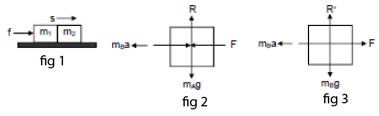F + mA a – f = 0

or F = f – mA a ..(1)

and

mB a – F = 0

=> F = mB a …(2)

From both the above equations,

f – mA a = mBa

or f = a (mA + mB )

or f = F/mB (mA + mB )

or f = F(1 + mA/mB)

[again using (2)]

Thus, the force exerted by the experimenter on A is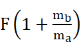Question 8: Raindrops of radius 1 mm and mass 4 mg are falling with a speed of 30 m/s on the head of a bald person. The drops splash on the head and come to rest. Assuming equivalently that the drops cover a distance equal to their radii on the head, estimate the force exerted by each drop on the head.

Solution:

Initial velocity = 0

Final velocity =30 m/s

Mass of the droplet= 4 mg = 4 x 10-6 kg

Radius of the droplet = 1mm = 10-3 m

Distance covered by the drop on the head, s = 10-3 m

Using the equation of motion

2as = v2 – u2

2a x 10-3 = 900

=> a = 45×103 m/s2

The force = F = ma = 1.8N

Question 9: A particle of mass 0.3 kg is subjected to a force F = – k x with k = 15 N/m. What will be its initial acceleration if it is released from a point x = 20 cm?

Solution:

Mass of the particle= 0.3 kg

Value of k = 15 N/m

x = 20 cm = 0.2 m

Now, Force = F = ma = kx

=> a = kx/m = 10 m/s2

Question 10: Both the springs shown in figure (5-E2) are unstretched. If the block is displaced by a distance x and released, what will be the initial acceleration?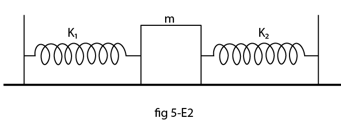Solution: The Block m towards left through displacement x.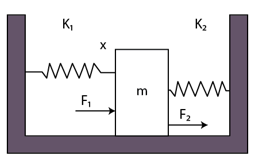Thus, the net force = F = k1x + k2x

Where k1 x force generate due to compression and force k2 x due to expandation.

=> F = (k1+k2)x

So, the acceleration = a = F/m = [(k1+k2)x]/m opposite to the displacement.

Question 11: A small block B is placed on another block A of mass 5 kg and length 20 cm. Initially the block B is near the right end of block A (figure 5. E3). A constant horizontal force of 10 N is applied to the block A. All the surfaces are assumed frictionless. Find the time elapsed before the block B separates from A.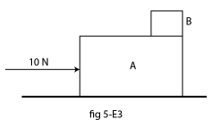Solution:

Initial velocity of small block = 0 m/s

Given, F = 10 and m = 5

Acceleration = a = F/m = 2 m/s2

Distance = s = 20 cm or 0.2 m

Using equation of motion, s = ut + (1/2) at2

=> 0.2 = (1/2) x 2 x t2

Or t = 0.45 s

Question 12: A man has fallen into a ditch of width d and two of his friends are slowly pulling him out using a light rope and two fixed pulleys as shown in figure (5-E4). Show that the force (assumed equal for both the friends) exerted by each friend on the road increases as the man moves up. Find the force when the man is at a depth h.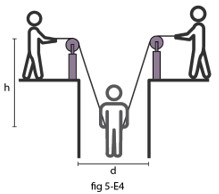Solution:

At the depth let the rope makes angle θ with the vertical.

From the free body diagram, we have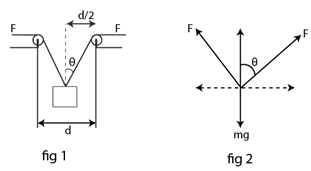F cos θ + F cos θ – mg = 0

=>F = mg/(2cos θ)

As the man moves up. θ increase means cos θ decreases. Thus, F increases.

When the man is at depth h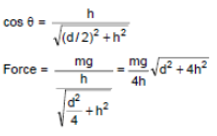Question 13: The elevator shown in figure (below) is descending with an acceleration of 2 m/s2. The mass of the block A is 0.5 kg. What force is exerted by the block A on the block B?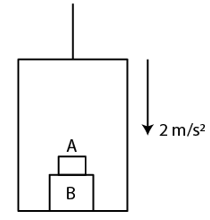Solution:

From the free body diagram,

The apparent weight of the box = 0.5 x 10-2×0.5 = 4N

Now, the reaction force on the box B due to A is = apparent weight of box A= 4N

Question 14: A pendulum bob of mass 50 g is suspended from the ceiling of an elevator. Find the tension in the string if the elevator (a) goes up with acceleration 1.2 m/s2, (b) goes up with deceleration 12 m/s2, (c) goes up with uniform velocity, (d) goes down with acceleration 1.2 m/s2, (e) goes down with deceleration 1.2 m/s2 and (f) goes down with uniform velocity.

Solution:

Mass of the bob = 50g or 0.05 kg

When the elevator is at rest the tension in the string weight of the bob = 9.8 x 50/1000 = 0.49N

a) When it goes up with an acceleration in upward direction a pseudo force acts on the bob in downward direction.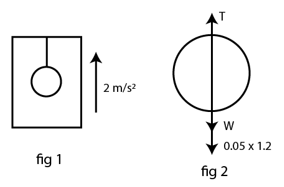The tension in the string becomes = T =0.49+0.05×1.2 = 0.55N

b) When it goes up with a deceleration in upward direction a pseudo force acts on the bob in downward direction with negative sign.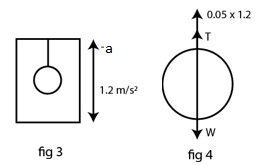The tension in the string becomes= T = 0.49-0.05×1.2=0.43N

c) When the elevator makes uniform motion: T – W = 0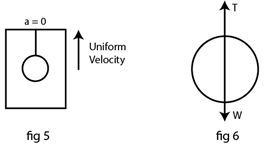The tension in the string becomes = T =0.05 x 9.8 = 0.49N

d) T + 0.05 x 1.2 – W = 0

=> The tension in the string becomes = T = 0.49 – (0.05 x 1.2 ) = 0.43 N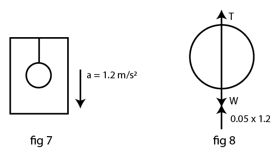e) When it goes down with a deceleration in downward direction a pseudo force acts on the bob in upnward direction with negative sign.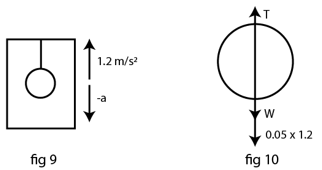The tension in the string becomes = T =0.49 + 0.05×1.2=0.55N

f) When it goes up with uniform velocity in downward direction no pseudo force.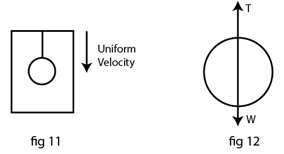In this case, uniform velocity acceleration = 0

T – W = 0

The tension in the string becomes = T = W = 0.49N

Question 15 : A person is standing on a weighing machine placed on the floor of an elevator. The elevator starts going up with some acceleration, moves with uniform velocity for a while and finally decelerates to stop. The maximum and the minimum weights recorded are 72 kg and 60 kg. Assuming that the magnitudes of the acceleration and the deceleration are the same, find (a) the true weight of the person and (b) the magnitude of the acceleration. Take g = 9.9 m/s2.

Solution:

Maximum weight = 72Kg

Minimum weight = 60 kg

When the elevator moves with acceleration, pseudo force acts on the person in the downward direction.

In this case the weight is maximum as measured by the weighing machine,

72×9.9=W+ma —–(1)

Where a = acceleration of the elevator.

When the elevator moves with deceleration, pseudo force acts on the person in the upward direction.

In this case the weight is minimum as measured by the weighing machine,

60×9.9=W-ma —–(2)

Adding (1) and (2) we get,

132×9.9 = 2mg = 2mx9.9

Thus, the weight W = 66 kg

Again, Subtracting (2) from (1)

12×9.9=2ma=2x66xa

Thus, the magnitude of acceleration = a = 0.9 m/s2

Question 16: Find the reading of the spring balance shown in figure (5-E6). The elevator is going up with an acceleration of g/10, the pulley and the string are light and the pulley is smooth.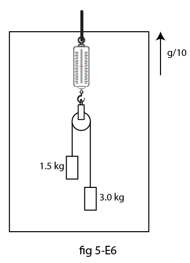Solution:

Mass of block A =1.5 kg

Mass of block B = 3 kg

From the free body diagram,

The tension in the string of block A = 1.5g + 11g/10 g N

The tension in the string of block A = 3g x 11g/10 N

Question 17: A block of 2 kg is suspended from the ceiling through a massless spring of spring constant k = 100 N/m. What is the elongation of the spring? If another 1 kg is added to the block, what would be the further elongation?

Solution:

Given:

Mass of the block = m = 2 kg

Spring constant = k = 100 N/m

The block is in equilibrium the force due to spring must be equal to the weight of the block

mg = kx ; Where x is the required extension,

on putting values, we get

=> x = 0.2 m

Now when 1 kg more is added to the block, the weight of the block increases to 30N (given)

Now, mg = kx

[Using the equation of equilibrium]

or x = 0.3 m

Again, Elongation = elongation due to 3kg – elongation due to 2kg

= 0.3-0.2 = 0.1m

Question 18: Suppose the ceiling in the previous problem is that of an elevator which is going up with an acceleration of 2 m/s2. Find the elongations.

Solution:

Acceleration of the elevator =a= 2 m/s2

Mass of the block =m= 2 kg

Apparent weight due to pseudo force = 23.6 N

In equilibrium, we have, apparent weight = kx

=> 23.6 = 100x

or x = 0.24 m

When 1kg body is added total mass, we have 3 kg

Now, Apparent weight of the block becomes = 2 x 9.8 + 3×2 + 1×98 = 35.4 N

The apparent weight=kx

=> 35.4 N=100x

Or x = 0.36m

Now as per the question we are asked to find further elongation,

Further elongation = elongation due to 3kg – elongation due to 2kg

= 0.36 – 0.24

= 0.12 m

Question 19: The force of buoyancy exerted by the atmosphere on a balloon is B in the upward direction and remains constant. The force of air resistance on the balloon acts opposite to the direction of velocity and is proportional to it. The balloon carries a mass M and is found to fall down near the earth’s surface with a constant velocity u. How much mass should be removed from the balloon so that it may rise with a constant velocity u?

Solution:

Let velocity in both cases is “u” and the Buoyancy force be “ B“.

The resistive force = ku, where k is proportionality constant.

Case I:

When balloon is falling down with constant velocity,

Then equation for equilibrium becomes,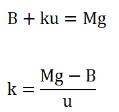Case II: For the balloon to rise with a constant velocity v (upward)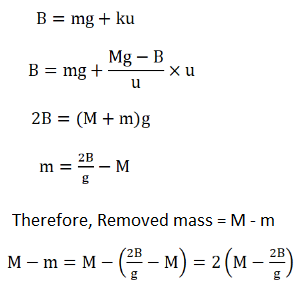Question 20: An empty plastic box of mass m is found to accelerate up at the rate of g/6 when placed deep inside water. How much sand should be put inside the box so that it may accelerate down at the rate of g/6?

Solution:

Mass = m

Case I: When it accelerates upward with acceleration “g/6”

Let B be the buoyant force, we have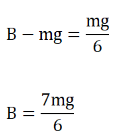Case II: When it accelerates downward with acceleration “g/6” after addition of sand of mass “s” kg.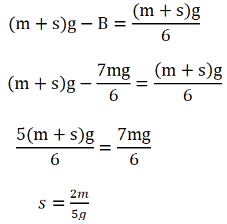Question 21: A force F = v x A is exerted on a particle in addition to the force of gravity, where vector v is the velocity of the particle and A is a constant vector in the horizontal direction. With what minimum speed a particle of mass m be projected so that it continues

to move undeflected with a constant velocity?

Solution:

For a particle to move undeflected with constant velocity, net force will be zero.

=> v x A + mg = 0

Velocity must be in direction perpendicular to plane in which gravity acts.

= Av sin θ, where θ is the angle between A vector and velocity of the particle.

Now, vxA = -mg

Or A v sinθ = -mg

Or v = -mg/Asin θ

Now, for the velocity to be minimum, sin θ must be maximum,

and maximum value of sinθ = 1

Minimum velocity of the particle = v = (-mg/A) m/s

Question 22: In a simple Atwood machine, two unequal masses m1 and m2 are connected by a string going over a clamped light smooth pulley. In a typical arrangement (figure below) m1 = 300 g and m2 = 600 g. The system is released from rest. (a) Find the distance travelled by the first block in the first two seconds. (b) Find the tension in the string, (c) Find the force exerted by the clamp on the pulley.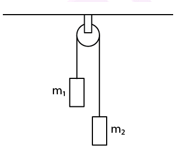Solution:

Mass m1 = 300 g or 0.3 kg

Mass m2 = 600 g or 0.6 kg

Let the mass 1 be moving upward and mass 2 moves downward

Equation for mass 1,

T – m1g = m1a —–(1)

Equation for mass 2,

m2g – T = m2a ——(2)

Adding (1) and (2), we get,

a = (m2-m1)/(m2+m1) g

Now putting the values, we get,

a = 1/3 g

a) Using the equation of motion, 2s = ut+ (1/2) at2

Where u=initial velocity = 0 m/s and tie t=2s

s = 6.65 m

b) From equation (1), we get,

T = m1(g+a)

= 0.3 x (13g+g)

= 0.4 g or 3.92 N

c) Force on the clamp is due to tension from both side of the pulley, i.e., twice of tension

Therefore, required force = F = 2T = 7.8N

Question 23: Consider the Atwood machine of the previous problem. The larger mass is stopped for a moment 2.0 s after the system is set into motion. Find the time elapsed before the string is tight again.

Solution:

When masses m1 and m2 are connected by a string passing over a pulley and when the pulley comes in motion, then mass m2 is stopped in 2 seconds.

Equation of motion:

T – m1 g = m1 a ……..(1)

m2 g − T = m2 a ………(2)

Solving (1) and (2), we have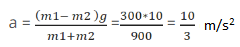In 2 sec the velocity acquired by mass m1 is given by,

Applying first equation of motion, we have

0 = v −10/4 × 2

or v = 20/3 m/s

Now, the time value with acceleration due to gravity is

20/3− 10 t or t = 2/3 sec

Question 24: Figure (below) shows a uniform rod of length 30 cm having a mass of 3.0 kg. The strings shown in the figure are pulled by constant forces of 20 N and 32 N. Find the force exerted by the 20 cm part of the rod on the 10 cm part. All the surfaces are smooth and the strings and the pulleys are light.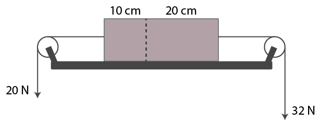Solution:

Here, m1= 3 kg × (10/30) = 1 kg

m2 = 3 kg × (20/30) = 2 kg

Force exerted by m1 on m2 = m1a = 1 x 4 = 4 N = Force exerted by m2 on m

Therefore, total force = 20 + 4 = 24 N

Question 25: Consider the situation shown in figure (5-E9). All the surfaces are frictionless and the string and the pulley are light. Find the magnitude of the acceleration of the two blocks.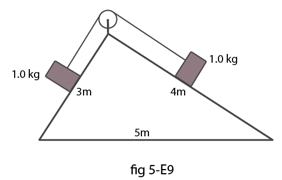Solution:

From the free body diagrams,

mg sinθ1 − T = ma ……..(1)

T – mg sinθ2 = ma …….(2)

mg(sinθ1− sinθ2) = 2ma

=> 2a = g (sinθ1 − sinθ2)

=> a = g/5 × 12 = g/10

Question 26: A constant force F = m2g/2 is applied on the block of mass m1 as shown in figure (below). The string and the pulley are light and the surface of the table is smooth. Find the acceleration of m1.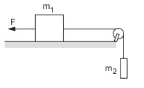Solution: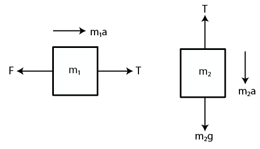From the free body diagram of block of mass m1,

m1a = T − F …………(1)

For block of mass m2,

m2a = m2g − T ……….(2)

Adding (1) and (2), we get:

a (m1 + m2) = m2g – F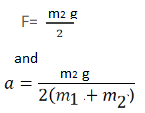The above equation is the acceleration of mass m1 towards the right.

Question 27: In figure (below) m1 = 5 kg, m2 = kg and F = 1 N. Find the acceleration of either block. Describe the motion of m if the string breaks but F continues to act.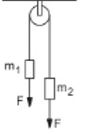Solution: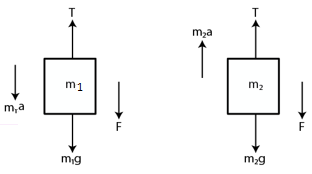m1 = 5 kg, m2 = 2 kg and F = 1 N

Let the acceleration of the blocks be a.

From the free-body diagram,

m1a = m1g + F − T ………..(1)

Again, m2a = T − m2g − F ………..(2)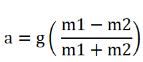=>a = 4.2 m/s2 = acceleration of the block

After the string breaks, m1 moves downward with force F acting downward. Then,

m1a = F + m1g

5a = 1 + 5g

or g = 0.2 m/s2

Question 28: Let m1 = 1 kg, m2 = 2 kg and m3 = 3 kg in figure (below). Find the accelerations of m1, m2 and m3. The string from the upper pulley to m1 is 20 cm when the system is released from rest. How long will it take before m1 strikes the pulley?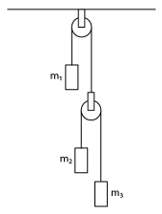Solution:

Let m1 = 1 kg, m2 = 2 kg and m3 = 3 kg (Given)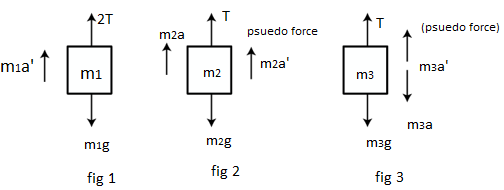Actual acceleration of the blocks m1, m2 and m3 will be: a1, (a1 − a2) and (a1 + a2)

From figure 2, T − g – a1 = 0 ……..(1)

From figure 3, T/2 -2g – 2(a1 – a2)=0 ….…(2)

From figure 4, T/2 – 3g – 3(a1 + a2 ) = 0 ………(3)

Eliminate T using equations (1) and (2),

g + a2 = 4g + 4 (a1 + a2)

5a2 − 4a1 = 3g ……..…(4)

From equations (2) and (3), we get:

2g + 2(a1 – a2) = 3g − 3 (a1 – a2)

5a1 + a2= g ………..(5)

Solving (4) and (5), we get

a1 = 2g/29 and a2 = g – 5a1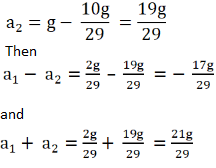So, accelerations of m1, m2 and m3 are 19g/29 up, 17g/29 down and 21g/29 down, respectively.

Now, u = 0, s = 20 cm = 0.2 m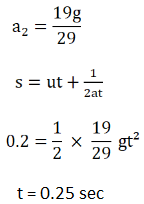Question 29: In the previous problem, suppose m2 = 2.0 kg and m3 = 3.0 kg. What should be the mass m so that it remains at rest?

Solution:

m1= 1kg, m2= 2kg, and m3 = 3kg

For m1 to be at rest, a1 = 0.

T − m1 g = 0

T = m1 g ……(1)

For mass m2, T/2 − 2g = 2a

T = 4a + 4g …….(2)

For mass m3, 3g –T/2 = 2a

T = 6g − 6a …(3)

From (2) and (3), we get:

3T – 12g = 12g – 2T

T = 24g/5 = 4.08g

Again (1) => we get: m1 = 4.8kg

Question 30: Calculate the tension in the string shown in figure (below). The pulley and the string are light and all surfaces are frictionless. Take g = 10 m/s2.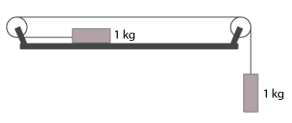Solution:

T + ma =mg ……..(1)

And T = ma ……..(2)

From (1) and (2), we get:

ma + ma = mg

=>2ma = g

=> a=g/2 =5 m/s2

And (2)=> T = ma = 5 N

Question 31: Consider the situation shown in figure (below). Both the pulleys and the string are light and all the surfaces are frictionless. (a) Find the acceleration of the mass M. (b) Find the tension in the string. (c) Calculate the force exerted by the clamp on the pulley A in the figure.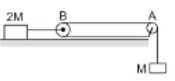Solution: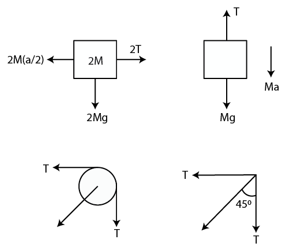Let the acceleration of mass M be “a”.

So, the acceleration of mass 2M will be “a/2”

(a) 2M(a/2) – 2T = 0

=> Ma = 2T

T + Ma − Mg = 0

=> Ma2+Ma=Mg

=> 3Ma=2Mg

or a = 2g/3

(b) Tension, T = Ma/2 = Mg/3

(c) Let T’ = resultant of tensions

T’ = √(T^2+T^2) = √2 T

T’=√2T = √(2Mg)/3

Again, tanθ= T/T = 1

or θ = 45°

So, the force exerted by the clamp on the pulley is √(2Mg)/3 at an angle of 45° with the horizontal.

Question 32: Find the acceleration of the block of mass M in the situation shown in figure (below). Ail the surfaces are frictionless and the pulleys and the string are light.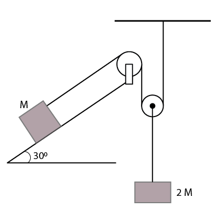Solution:

Let acceleration of the block of mass 2M be a.

So, acceleration of the block of mass M will be 2a.

M(2a) + Mg sinθ − T = 0

=> T = 2Ma + Mg sinθ ………(1)

2T + 2Ma − 2Mg = 0

From (1)

2(2Ma + Mg sinθ) + 2Ma − 2Mg = 0

4Ma + 2Mg sinθ + 2Ma − Mg = 0

6Ma + 2Mgsin30° + 2Mg = 0

6Ma = Mg

=> a = g/6

Hence, the acceleration of mass, M = 2a = 2g/6 = g/3